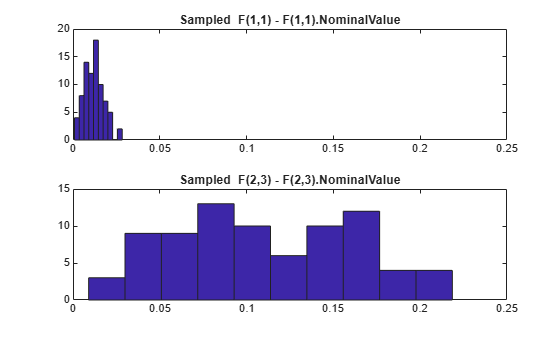# ucomplexm

Create uncertain complex matrix

## Syntax

```M = ucomplexm('Name',NominalValue)
M = ucomplexm('Name',NominalValue,'WL',WLvalue,'WR',WRvalue)
M = ucomplexm('Name',NominalValue,'Property',Value)
```

## Description

`M = ucomplexm('Name',NominalValue)` creates an uncertain complex matrix representing a ball of complex-valued matrices, centered at a `NominalValue` and named `Name`.

`M = ucomplexm('Name',NominalValue,'WL',WLvalue,'WR',WRvalue)` creates an uncertain complex matrix with weights `WL` and `WR`. Specifically, the values represented by `M` are all matrices `H` that satisfy `norm(inv(M.WL)*(H - M.NominalValue)*inv(M.WR)) <= 1. WL` and `WR` are square, invertible, and weighting matrices that quantify the size and shape of the ball of matrices represented by this object. The default values for `WL` and `WR` are identity matrices of appropriate dimensions.

Trailing Property/Value pairs are allowed, as in

```M = ucomplexm('NAME',nominalvalue,'P1',V1,'P2',V2,...) ```

The property `AutoSimplify` controls how expressions involving the uncertain matrix are simplified. Its default value is` 'basic'`, which means elementary methods of simplification are applied as operations are completed. Other values for `AutoSimplify` are` 'off''`, no simplification performed, and` 'full'` which applies model-reduction-like techniques to the uncertain object.

## Examples

collapse all

Create a `ucomplexm` with the name `F`, nominal value `[1 2 3; 4 5 6]`, and weighting matrices `WL = diag([.1.3])`, `WR = diag([.4 .8 1.2])`.

```F = ucomplexm('F',[1 2 3;4 5 6],'WL',diag([.1 .3]),... 'WR',diag([.4 .8 1.2]))```
```F = Uncertain complex matrix "F" with 2 rows and 3 columns. ```

Sample the difference between the uncertain matrix and its nominal value at 80 points, yielding a 2-by-3-by-80 matrix `typicaldev`.

`typicaldev = usample(F - F.NominalValue,80);`

Plot histograms of the deviations in the (1,1) entry and the (2,3) entry of the complex matrix.

The absolute values of the (1,1) entry and the (2,3) entry are shown by histogram plots. Typical deviations in the (1,1) entry should be about 10 times smaller than the typical deviations in the (2,3) entry.

```subplot(2,1,1); td11 = squeeze(typicaldev(1,1,:)); hist(abs(td11)); xlim([0 .25]) title('Sampled F(1,1) - F(1,1).NominalValue') subplot(2,1,2); td23 = squeeze(typicaldev(2,3,:)); hist(abs(td23)); title('Sampled F(2,3) - F(2,3).NominalValue')```